说明

• 本页面无手机端适配，强制缩放阅读。
• 使用纯html格式，保存教学用ppt，添加了部分个人笔记。
• 目录工作正常，可以跳转。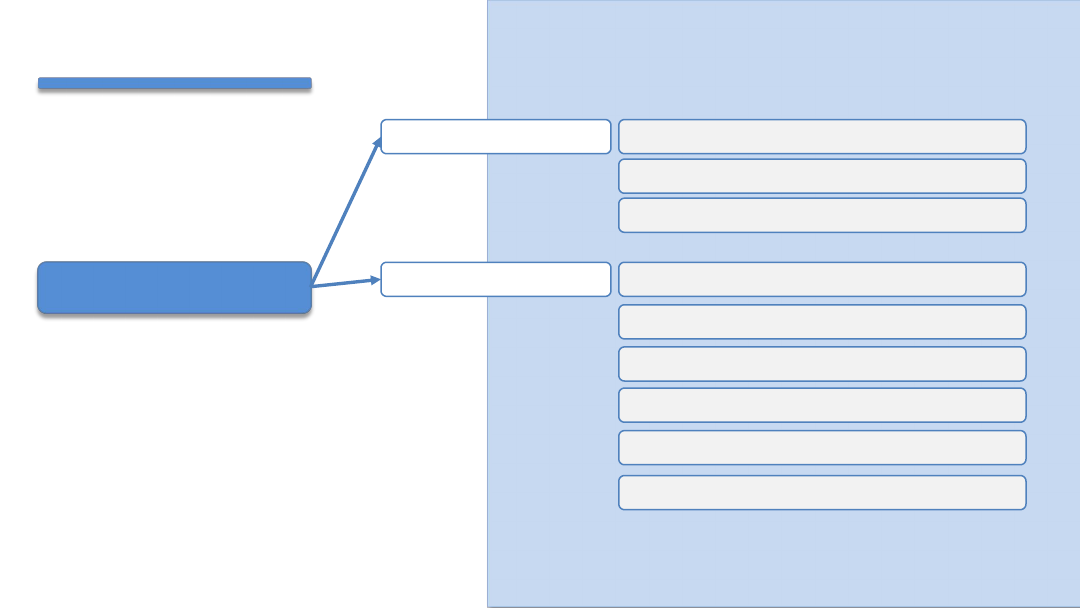损失函数• 损失函数(Loss Function)，也有称之为代价函数(Cost Function)，• 度量决策函数f(x)和实际值之间的差异。
• 作为模型性能参考。损失函数值越小，说明预测输出和实际结果(也称期望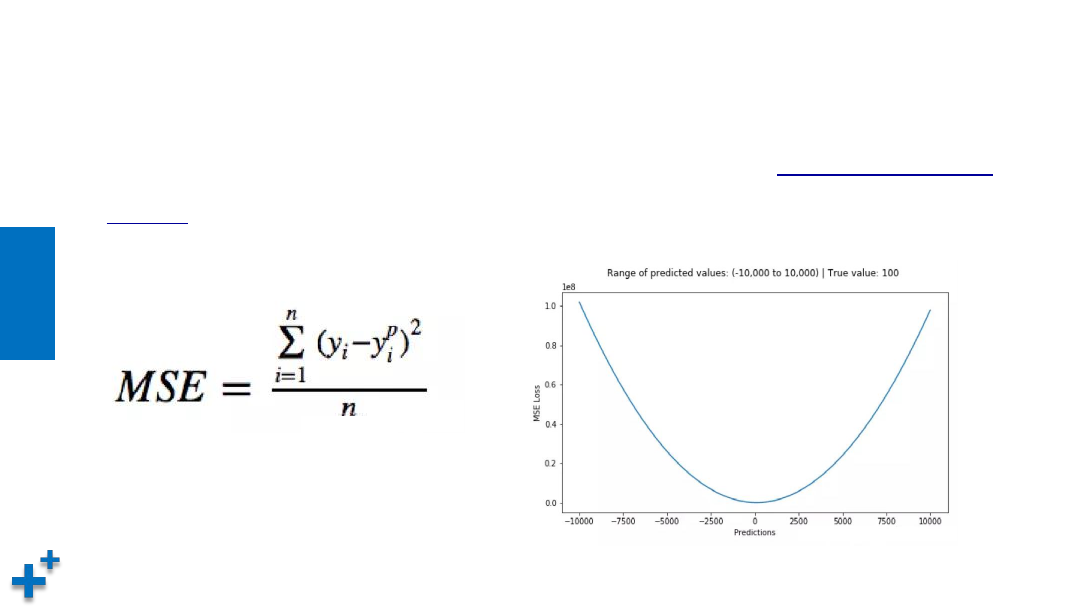• 均方误差(Mean square error)损失函数。均方误差是

，它是预测值与目标值之间差值的平方和，其公式和图像如下所示：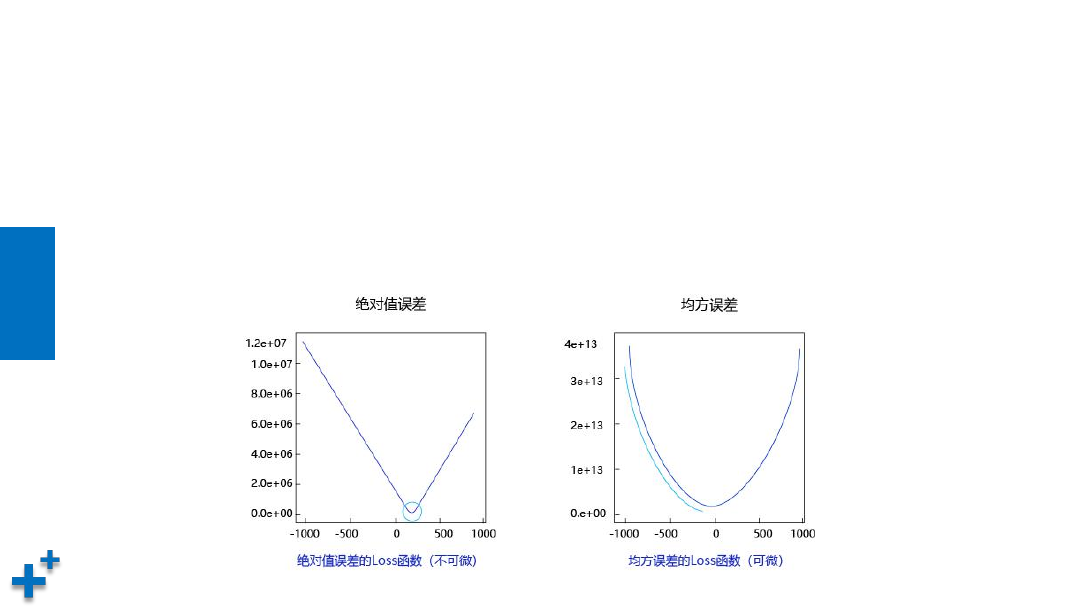• 曲线的最低点是可导的
• 越接近最低点，曲线的坡度逐渐放缓，有助于通过当前的梯度来判断接近最低点的程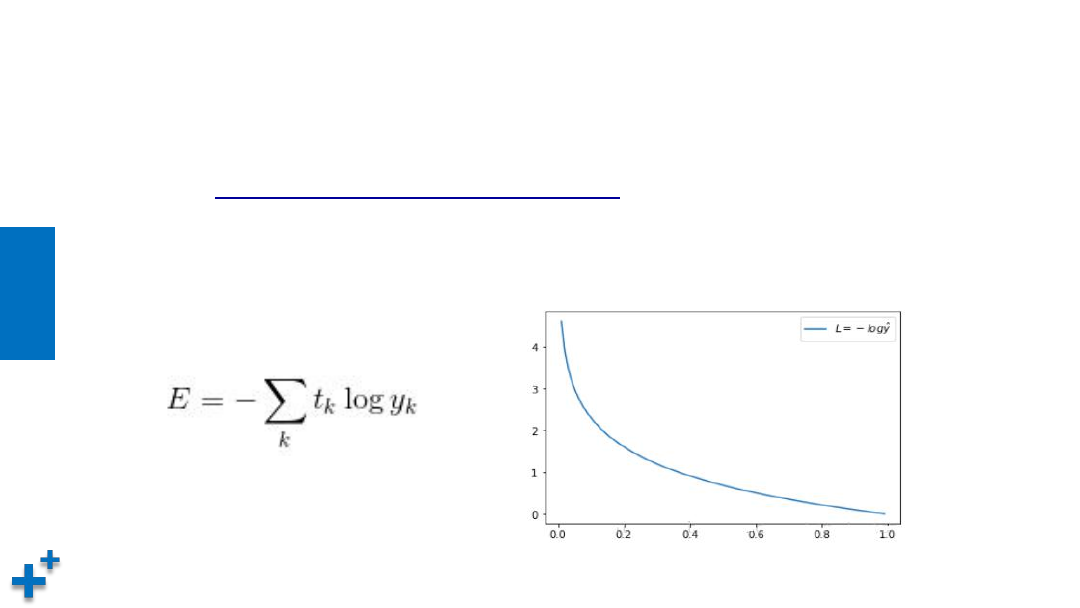• 交叉熵(Cross Entropy)。交叉熵是Shannon信息论中一个重要概念，

，在机器学习中用来作为分类

k

,其交叉熵函数公式及图形

梯度下降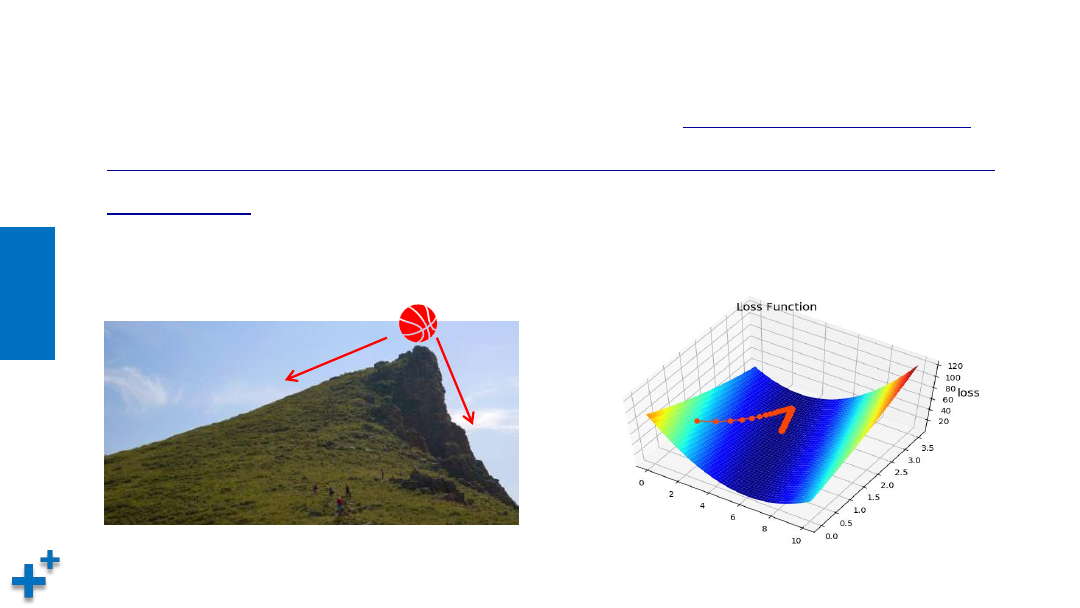• 通过损失函数，我们将“寻找最优参数”问题，转换为了“寻找损失函数

(1)损失是否足够小？如果不是，计算损失函数的梯度。
(2)按梯度的反方向走一小步，以缩小损失。
(3)循环到(1)。

”。通过这样的方法，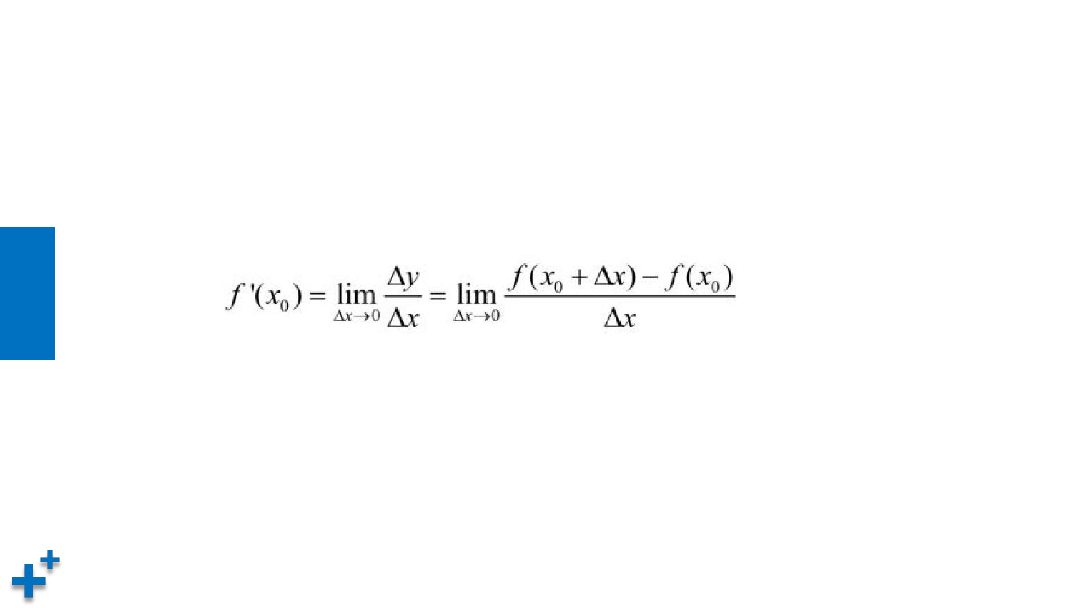• 导数的定义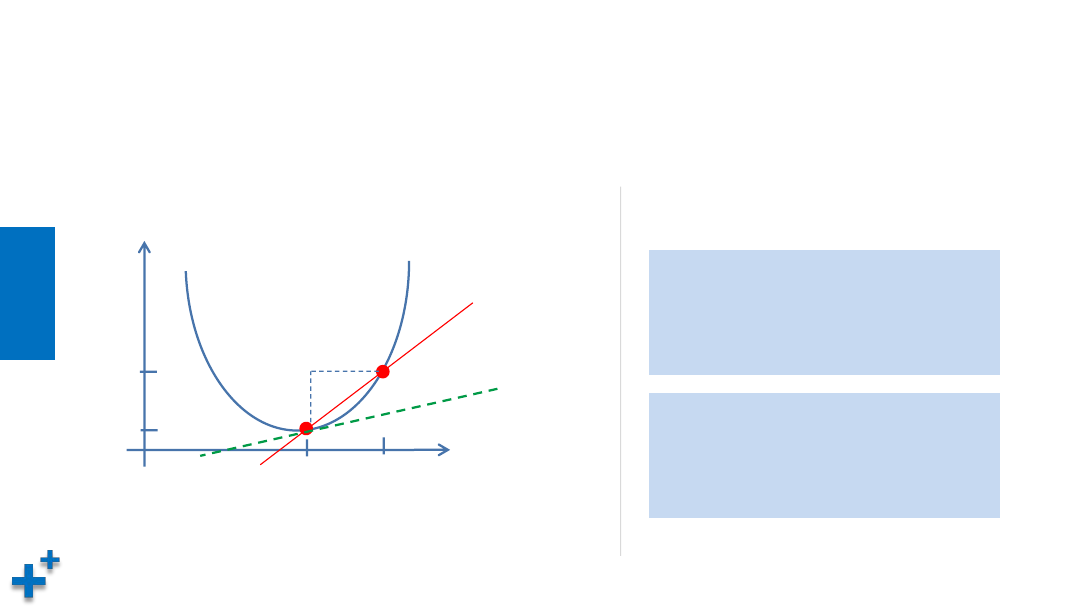• 导数的含义：反映变化的剧烈程度(变化率)

x

y

x

0

x

1

Δx

Δy

y

0

y

1

2

+ 1

x

0

=1                  y

0

=2

x

1

= 1.0001        y

1

=2.00020001

∆y / ∆x ≈   0.0002 / 0.0001 = 2

x

0

=2                  y

0

=5

x

1

= 2.0001        y

1

=5.00040001

∆y / ∆x ≈   0.0004 / 0.0001 = 4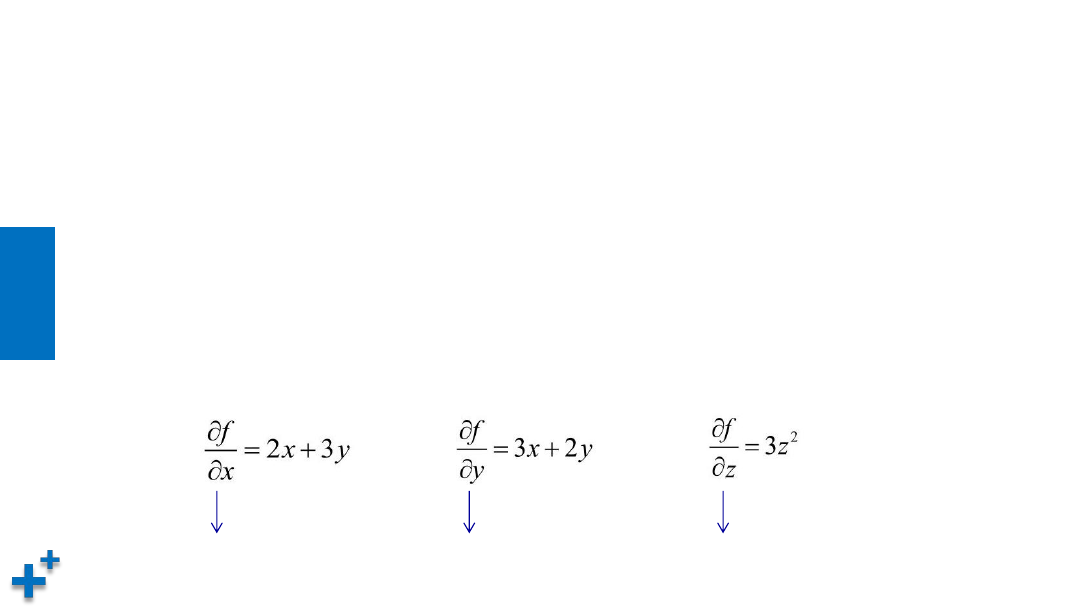• 偏导数

“偏导”的英文本意是“partial derivatives“(表示局部导数)。对于多维变量函数

(相比于全部变量，这里只求一个变量，即为“局部”)。例如有函数：

f = x

+ 3xy + y

+ z

3

y, z为常量

x, z为常量

x, y为常量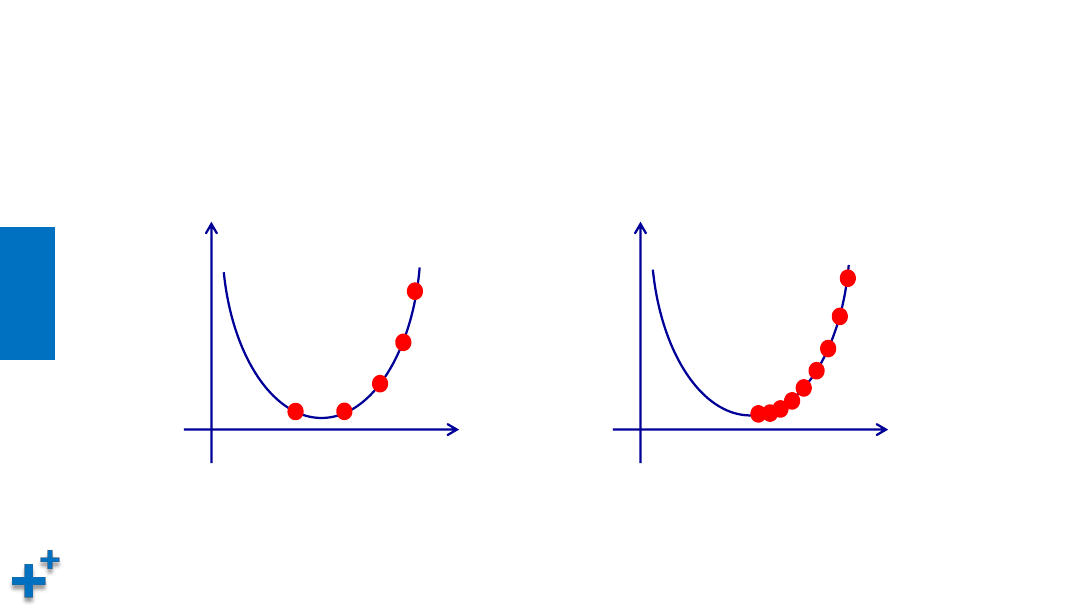• 学习率是梯度下降过程中，在梯度值前面的系数，用来控制调整的步幅大小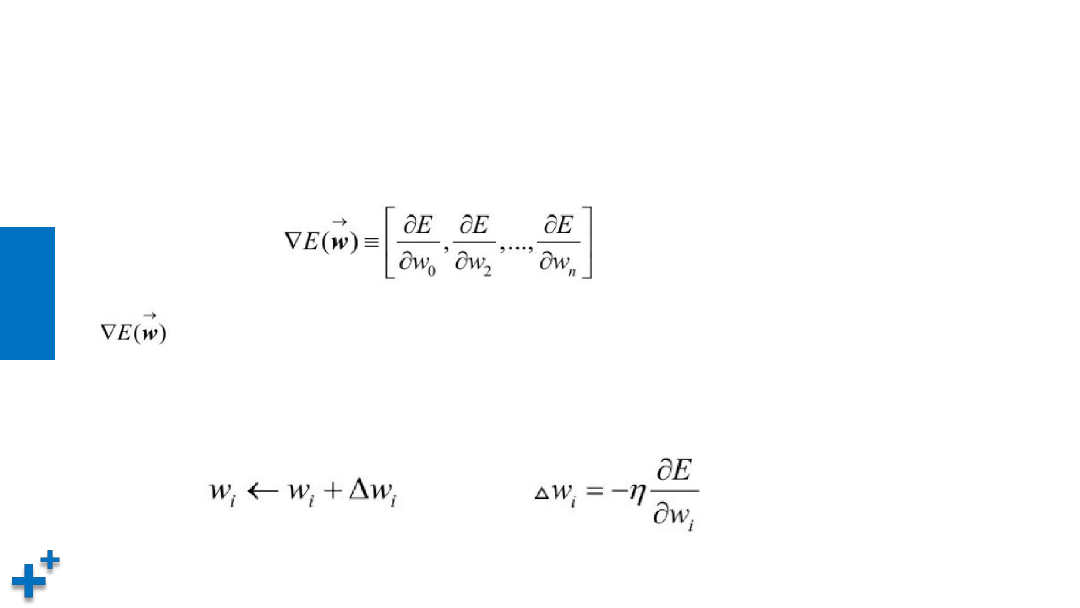• 神经网络中的权值参数是非常多的，因此针对损失函数E的权值向量的梯度如以下公

表示损失函数E的梯度，它本身也是一个向量，它的多个维度分别由损失函数
E对多个权值参数w

i• 批量梯度下降

Ø

ü

ü

Ø

ü• 随机梯度下降

Ø

ü

Ø

ü

ü

ü• 小批量梯度下降

Ø

ü

ü

Ø

ü

batch_size的不当选择可能会带来一些问题。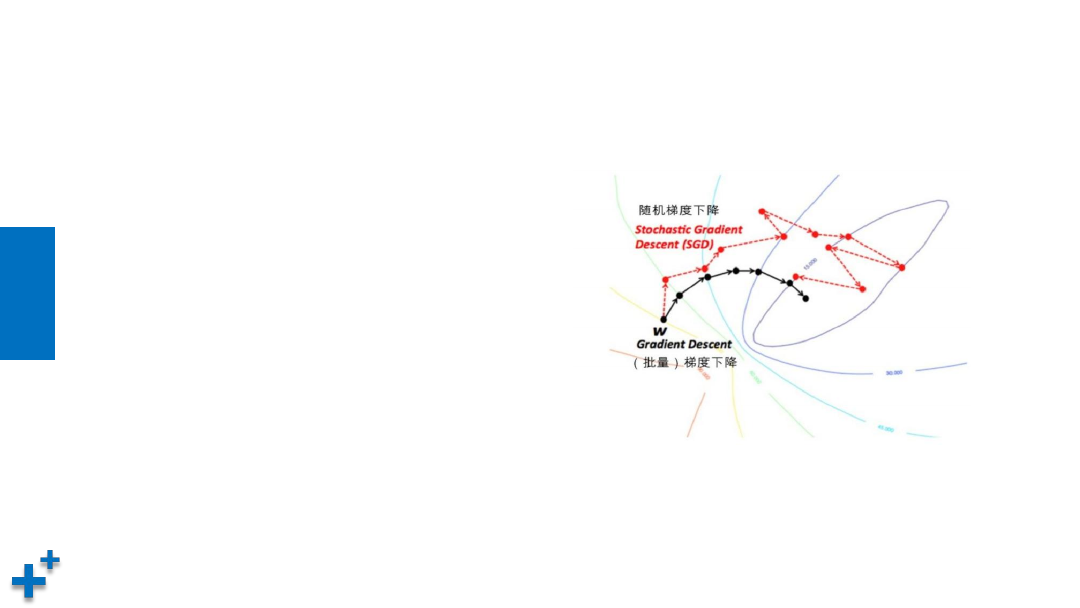• 几种梯度下降算法收敛比较

Ø

Ø

Ø• 本章节介绍了损失函数与梯度下降概念与算法

ü

ü